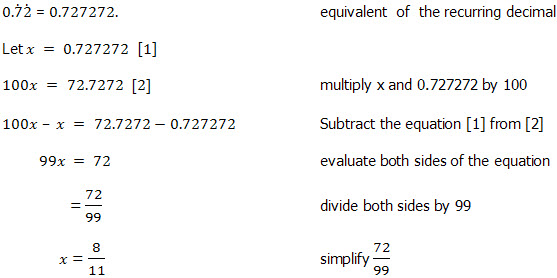# Geometric Progression, Series & Sums

## Introduction

A geometric sequence is a sequence such that any element after the first is obtained by multiplying the preceding element by a constant called the common ratio which is denoted by r. The common ratio (r) is obtained by dividing any term by the preceding term, i.e.,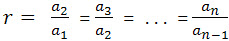where r common ratio a1 first term a2 second term a3 third term an-1 the term before the n th term an the n th term

The geometric sequence is sometimes called the geometric progression or GP, for short.

For example, the sequence 1, 3, 9, 27, 81 is a geometric sequence. Note that after the first term, the next term is obtained by multiplying the preceding element by 3.

The geometric sequence has its sequence formation:To find the nth term of a geometric sequence we use the formula: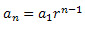where r common ratio a1 first term an-1 the term before the n th term n number of terms

## Sum of Terms in a Geometric Progression

Finding the sum of terms in a geometric progression is easily obtained by applying the formulas:

nth partial sum of a geometric sequence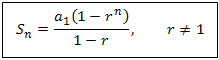sum to infinity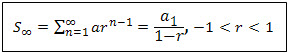where Sn sum of GP with n terms S∞ sum of GP with infinitely many terms a1 the first term r common ratio n number of terms

## Examples of Common Problems to Solve

Write down a specific term in a Geometric Progression

Question

Write down the 8th term in the Geometric Progression 1, 3, 9, ...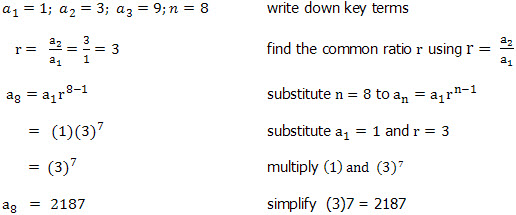Finding the number of terms in a Geometric Progression

Question

Find the number of terms in the geometric progression 6, 12, 24, ..., 1536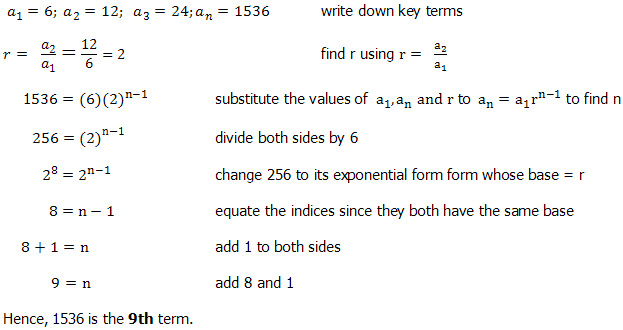Finding the sum of a Geometric Series

Question

 Find the sum of each of the geometric series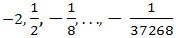Finding the sum of a Geometric Series to Infinity

Question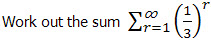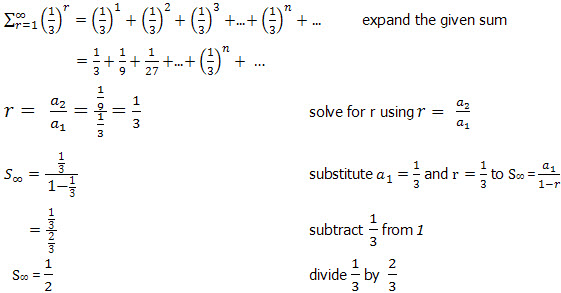Converting a Recurring Decimal to a Fraction

Decimals that occurs in repetition infinitely or are repeated in period are called recurring decimals.

For example, 0.22222222... is a recurring decimal because the number 2 is repeated infinitely.

The recurring decimal 0.22222222... can be written as.

Another example is 0.234523452345... is a recurring decimal because the number 2345 is repeated periodically.

Thus, it can be written as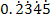or it can also be expressed in fractions.

Question

Expressas a fraction in their lowest terms.